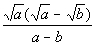# Intermediate Algebra Tutorial 41

Intermediate Algebra
Tutorial 41: Rationalizing Denominators and NumeratorsAnswer/Discussion to 1a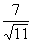Step 1: Multiply numerator and denominator by a radical that will get rid of the radical in the denominator.

Since we have a square root in the denominator,  we need to multiply by the square root of an expression that will give us a perfect square under the square root in the denominator.

So in this case, we can accomplish this by multiplying top and bottom by the square root of 11: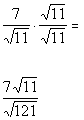*Mult. num. and den. by sq. root of 11

*Den. now has a perfect square under sq. root

AND

Step 3: Simplify the fraction if needed.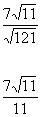*Sq. root of 121 is 11Answer/Discussion to 2a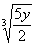Step 1: Multiply numerator and denominator by a radical that will get rid of the radical in the numerator.

Since we have a cube root in the numerator,  we need to multiply by the cube root of an expression that will give us a perfect cube under the cube root in the numerator.

So in this case, we can accomplish this by multiplying top and bottom by the cube root of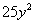: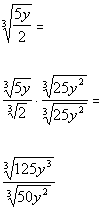*Mult. num. and den. by cube root of*Num. now has a perfect cube under cube root

AND

Step 3: Simplify the fraction if needed.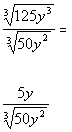*Cube root of 125 y cubed is 5yAnswer/Discussion to 3a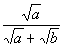Step 1: Find the conjugate of the denominator.

In general the conjugate of a + b is a - b and vice versa.

So what would the conjugate of our denominator be?

It looks like the conjugate is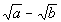.

Step 2: Multiply the numerator and the denominator of the fraction by the conjugate found in Step 1 .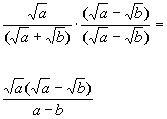*Mult. num. and den. by conjugate of den.
*Product of the sum and diff. of two terms

AND

Step 4: Simplify the fraction if needed.

No simplifying can be done on this problem so the final answer is: